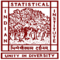Difference between Median and Average

# Difference between Median and Average

clickHere
Updated on Mar 6, 2023 15:29 IST

Average and median are two basic terms that are used in statistics very often. Median is the middle value in a set, whereas average is an arithmetic mean of set of numbers.

Median and Average are two essential terms that are used in statistics, and they are used very often. In this article, we will discuss Median vs. Average. The fundamental difference between Median and Average is that the Median is the middle value, whereas the Average is the arithmetic mean of values.

## What is the Median?

Definition

In any given set, median is the middle value of that dataset when they are sorted (either ascending or descending).

• It separates the set in two equal halves.
• Median is not much sensitive to the outliers.

Formula

Let there are n elements in any set, then

• When n is odd:

Median = ((n + 1)/2) th term

• When n is even:

Median = ( (n/2) th term + (n/2 + 1) th term) / 2

Now, let’s take some example to know how these formula can be used:

Example

1. Find the median of {3, 6, 5, 11, 8, 5, 7, 3, 9}

The given set has 9 elements, and the elements are not sorted, so let’s arrange them in ascending order.

{3, 3, 5, 5, 6, 7, 8, 9, 11}

Since, number of element is 9

Hence, Median = ((9 + 1)/2)th element = 5th element = 6

therefore, Median value of the given set is 6.

1. Find the median of {2, 2, 5, 8, 11, 150}

Elements of the given set are already sorted, and the number of element = 6

Hence, Median =( (6/2)th term + ((6/2) + 1)th term )/2 = (3rd term + 4th term)/2 = (5 + 8)/2 = 6.5

therefore, Median value of the given set is 6.5.

## What is an Average?

Definition

Average is defined as the mean value, equals to

sum of all observation / number of observation

• Average is also known as Arithmetic Mean
• It is highly sensitive to the outliers
• Mainly, used to find the central tendency of a normal distribution
• For a Normal Distribution
• Mean = Median = Mode

Formula

Let there are n elements in any set, then:

Average =sum of data values / number of data values =  (x1 + x2 + x3 + x4 + ……. + xn) / n

Example

1. Find the average of {3, 6, 5, 11, 8, 5, 7, 3, 9}.

Average = (3 + 6 +  5 + 11 + 8 + 5 + 7 + 3 + 9) / 9 = 57 / 9 = 6.34

Therefore, Average = 6.34

1. Find the median of {2, 2, 5, 8, 11, 150}.

Average = (2 + 2 + 5 + 8 + 11 + 150) / 6 = 178 /6 = 29.67

Therefore, Average = 29.67

Read Also: Introduction to Sampling and Resampling

## Key differences and similarities between Median and Average

• Average and Median both are “Measures of Central Tendency”.
• Average is calculated by adding up all the individuals and dividing by total number of observations, whereas median is calculated by choosing the “middle value” after arranging all the numbers either in ascending or descending order.
• Median finds the central tendency of skewed data, whereas Average is used to find the central tendency of normal distribution.
• Median is no much sensitive to the outlier, whereas Average is highly sensitive to the outliers.

## Conclusion

In this article, we have briefly discussed median vs average with examples.

Hope, you will like the article.

Keep Learning!!

Keep Sharing!!

clickHere

Vikram has a Postgraduate degree in Applied Mathematics, with a keen interest in Data Science and Machine Learning. He has experience of 2+ years in content creation in Mathematics, Statistics, Data Science, and Mac... Read Full Bio

## Trending Data Science Courses## Top Picks & New Arrivals